Function Repository Resource:

# CountWolframModelRules

Count canonical Wolfram model rules with a particular signature

Contributed by: Ed Pegg
 ResourceFunction["CountWolframModelRules"][signature,s] counts canonical Wolfram model rules with the specified signature, and s possible symbols.

## Examples

### Basic Examples (3)

Count the number of rules matching a simple signature and two symbols:

 In:=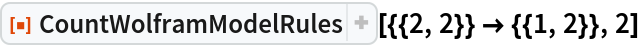Out=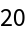Changing the order of the rule does not change the number of rules:

 In:=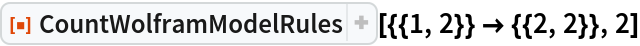Out=Count the number of rules matching more complex signatures and larger symbol counts:

 In:=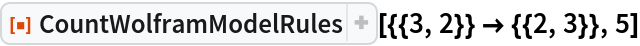Out=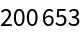In:=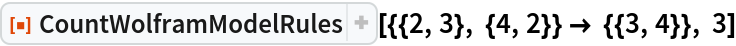Out=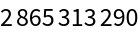In:=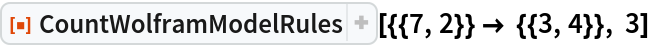Out=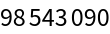### Scope (3)

#### Binary rule counts (1)

Create a table showing binary rule counts for many different signatures:

 In:=In:=Out=#### Ternary rule counts (1)

Create a table showing ternary rule counts for many different signatures:

 In:=In:=Out=#### 4-ary rule counts (1)

Create a table showing 4-ary rule counts for many different signatures:

 In:=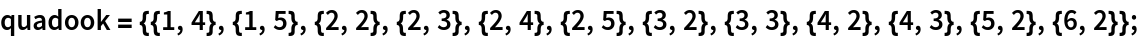In:=Out=## Version History

• 1.0.0 – 13 December 2019